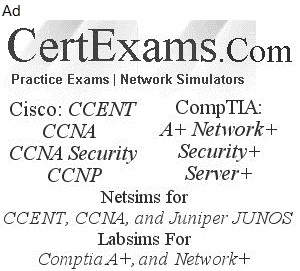# Cisco® CCENT Exam Cram Notes : Ipv4 Addressing

## 1. Networking Fundamentals

#### 4. IPv4 Addressing

4.3 Examples on subnetting:

Example 1 : You have an IP address of 156.16.3.47 with an 11-bit subnet mask. What are your valid hosts?

156.16.3.47 is a class B IP address and is normally used with a subnet mask of type 255.255.x.x

11 bit subnet mask is equivalent to 255.224.0.0=11111111.11100000.00000000.00000000

Wild card mask - 0.31.255.255

Host Min: 156.0.0.1

Host max: 156.31.255.254

Host/Network-2097150

Example 2: What is the subnet Id of the IP address 165.212.18.5/20?

The subnet Id of the IP address 165.212.18.5/20 is 165.212.16.0 The available host address range is 165.212.16.1 - 165.212.31.254 Broadcast address: 165.212.31.255

Example 3: You have a network ID of 168.8.0.0. You need to divide it into multiple subnets with at least 500 hosts per each subnet. Which subnet mask should you use so that you will be able to divide the network into maximum number of subnets?

I. Find the Class of the IP address, in this case it is a class B network. Class B network has the form N.N.H.H. Therefore, we have a total of 16 bits (two octets) for assigning to internal networks and hosts. The minimum number of host addresses required is 500 (see the question). The last octet corresponds to 2^8 = 256 hosts which is still less than 500 Hosts.. Therefore, you have to borrow one more bit from the third octet to make it 256*2 = 512 Hosts. This leaves 7 bits in the third octet for assigning subnet addresses. This is equal to 2^7=128 subnets.

II. Write the 7 bits available for subnetting in third octet in the form 11111110 (last bit being the Host bit). The decimal equivalent of the first seven bits is 2^7+2^6+2^5+2^4+2^3+2^2+2^1= 128 + 64 +32 + 16 + 8 + 4 + 2 = 254.

III. Now the subnet mask required is 255.255.254.0.

Example 4: You have an IP address 156.233.42.56 with a subnet mask of 7 bits. How many hosts and subnets are possible?

Class B network has the form N.N.H.H, the default subnet mask is 16 bits long. There is additional subnet mask of 7 bits long.

7 bits of subnet mask corresponds to (2^7)=128 subnets.

Some times, the subnet mask is specified with the bits available in the default subnet mask. In this case the bits available in default subnet mask is 16. Therefore, total number of bits available in the subnet mask are 16+7=23. If you are given a subnet mask of 23 bits long for a class B address, it is understood that it contains the bits from the default subnet mask as well.

9 bits (16-7) of host addresses corresponds to (2^9-2)=512-2 = 510 hosts.

Note that host IPs corresponding to all zeros and all ones cannot be used.

Example 5: You have a network address of 196.202.56.0 with four subnets. You want to allow for maximum number of Hosts. What is the subnet mask you need to apply?

We want to implement maximum possible Hosts. Therefore, we need to minimize the number of subnets. This minimum number is 4 here. If we reserve 2 bits, it results in 2^2=4 subnets. This is now optimized for maximum number of Hosts (as we have optimized for minimum number of subnets).

Write the 2 bits available for subnetting in fourth octet in the form 11000000 (Six 0s being Host bits). The decimal equivalent is 2^7+2^6= 128 + 64 = 192. , Now the subnet mask required is 255.255.255.192

Example 6: You have a network address of 196.202.56.0 with five Hosts on each subnet. You want to allow for maximum number of subnets. What is the subnet mask you need to apply?

I. The number of hosts required are five. We need to add Hosts of all ones and all zeros to this. This is because all zeros and all ones subnets belong to "this Host" and "all Hosts" broadcasts and can not be used. Therefore, the total number of Hosts addresses to be reserved is 5+2 = 7.

II. We want to implement maximum possible subnets. Therefore, we need to minimize the number of Hosts. This minimum number is 7 here. If we reserve 2 bits, it results in only 2^2=4 Hosts which is less than 7. Therefore, we have to reserve 3 bits for implementing Hosts, resulting in 2^3=8 Host addresses. This is now optimized for maximum number of Subnets (as we have optimized for minimum number of Hosts). This also leaves us with 5 bits in the fourth Octet for implementing subnets.

III. Write the 5 bits available for subnetting in fourth octet in the form 11111000 (Five 1s being subnet bits). The decimal equivalent is 2^7+2^6+2^5+2^4+2^3= 128 + 64 +32 + 16 + 8 = 248.

IV. Now the subnet mask required is 255.255.255.248.

Example 7: You have a router with two ports, each port with a distinct IP address. Both ports are connected to two distinct subnets (segments). The first subnet has 12 clients and a server. The second subnet has 14 clients and a server. What is the total number of IP addresses required?

The number of distinct IPs required are

1) One each per client computer

2) One each per server computer

3) One each per router interface.

There are 2 servers + 26 clients + 2 router interfaces. Therefore, the total number of IP addresses required are 30.

CCENT Cram Notes Contents